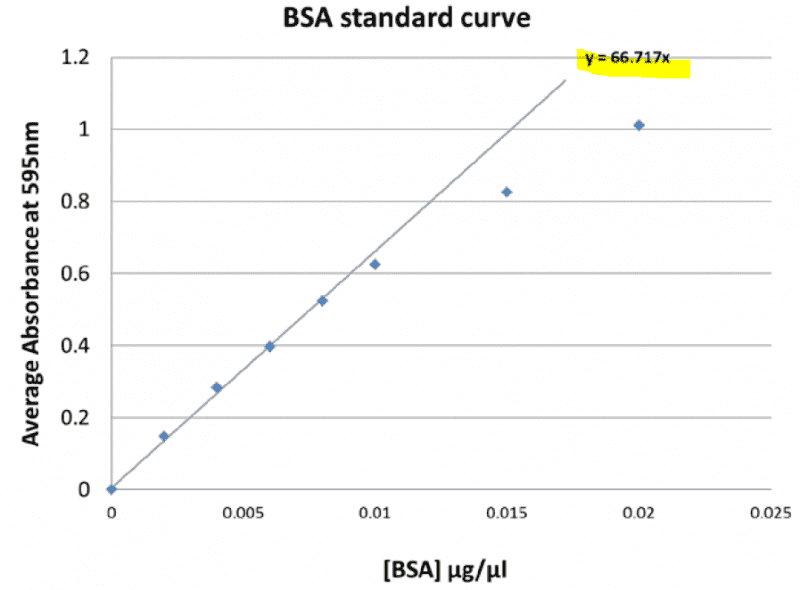tvtokyo

## Homework Statement

Hi, I have one more question about the Bradford Assay. This one involves dilution.

Given 1ml of protein A, I am to determine its protein concentration (in mg/ml) using Bradford Assay (with known BSA protein standards).
Also, Under a 5 times dilution, the average absorbance is 0.497.

## Homework Equations

The standard curve I obtained is:## The Attempt at a Solution

From the standard curve,
y = 66.717x
With absorbance = 0.497
0.497 = 66.717x
x = 0.00744 ug/ul
Since there is a dilution factor of 5
New x = 0.00744 * 5 = 0.0372 ug/ul = 0.0372 mg/ml ?? (is this correct?)

However, the correct answer is 0.372 mg/ml . What did I did wrongly??

## Answers and Replies

Homework Helper
Gold Member
x = 0.00744 ug/ul
Looks good.
New x = 0.00744 * 5 = 0.0372 ug/ul = 0.0372 mg/ml ??
Looks good.

Answer keys in textbooks are "edited/proofed" by "slave labor," aka grad students and post-docs. Often, "half" changes are made after proofing --- problem statements change, and answers don't.

Mentor
In your other question you clearly ignored part of the information given, hard to comment on this one not seeing the whole problem.